Open in App
Not now

# Lattice Graphs in R

• Last Updated : 30 Jan, 2023

Lattice graphs in R are a type of graphical representation that uses a grid-like structure to display data. They are commonly used in statistics and data visualization to show the relationship between multiple variables. In R Programming Language, lattice graphs can be created using the lattice package, which provides a variety of functions for creating different types of lattice plots such as scatter plots, density plots, and bivariate plots. These plots are highly customizable and can be used to create detailed and informative visualizations of complex data sets.

In R, the package “lattice” provides functions for creating and plotting lattice graphs. These functions include xyplot(), bwplot(), and wireframe(), which can be used to create scatterplots, boxplots, and 3D surface plots respectively. The package also includes the function levelplot() which can be used to create heat maps. Lattice graphs can be customized by adjusting the scales, colors, and other graphical parameters.

In addition, the package “ggplot2” also provides functions for creating lattice-based plots, such as geom_tile() for creating heat maps, and geom_raster() for creating raster plots.

## R

 `library``(lattice)``x <- 1:10``y <- x^2``xyplot``(y ~ x, type = ``"l"``)`

Output: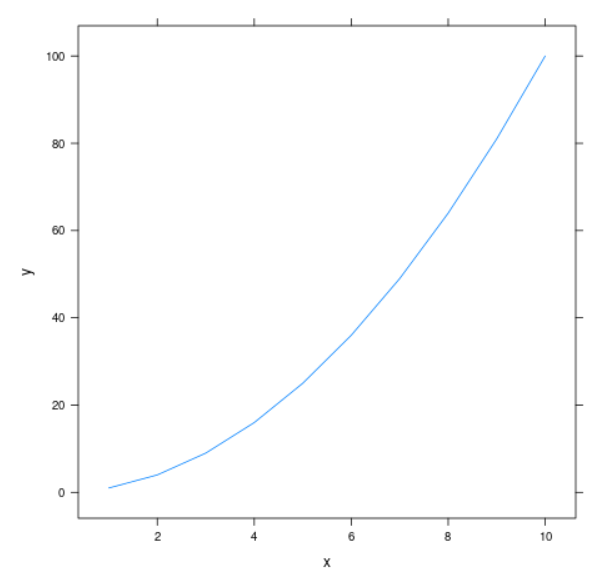## R

 `library``(lattice)``x <- 1:10``y <- 1:10``z <- ``rnorm``(100)``cloud``(z ~ x*y, main = ``"3D Lattice Graph"``)`

Output: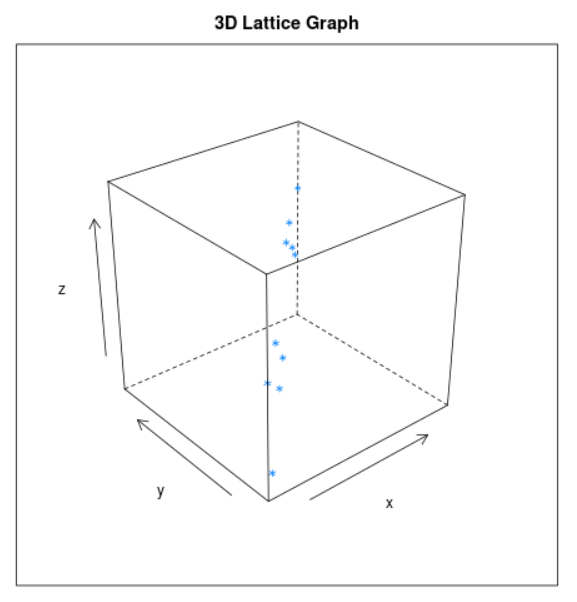### Level Plot

A level plot is a type of lattice graph that is used to display the values of a function of two variables over a rectangular region. In R, the lattice package can be used to create level plots using the levelplot() function.

Example: Creating a level plot of a 2-dimensional function using the lattice package in R

## R

 `library``(lattice)``x <- ``seq``(-``pi``, ``pi``, length.out = 100)``y <- ``seq``(-``pi``, ``pi``, length.out = 100)``z <- ``outer``(x, y, ``function``(x, y) ``sin``(``sqrt``(x^2 + y^2)))``levelplot``(z, xlab = ``"x"``, ylab = ``"y"``,``          ``main = ``"2D Sin Function"``)`

Output: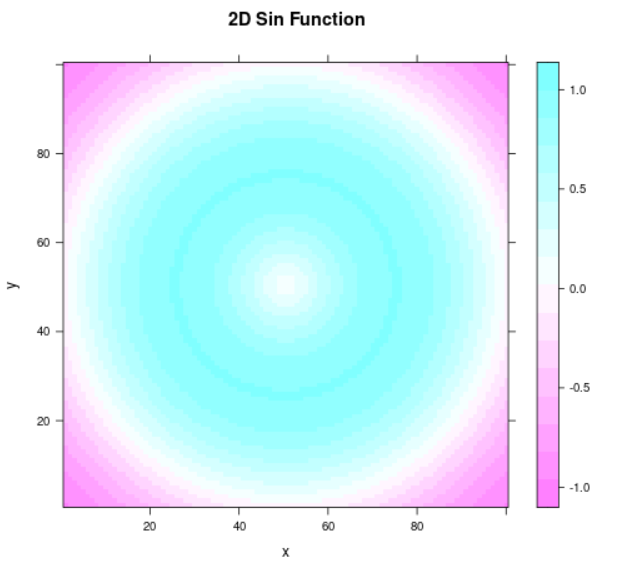### Trellis Graph

A trellis graph is a type of lattice graph that shows multiple plots arranged in a grid pattern. Each plot represents a different subset of the data. For example, in R, we can create a trellis graph using the “lattice” package and the “xyplot” function. Here is an example:

## R

 `library``(lattice)``data``(mtcars)``xyplot``(mpg ~ hp | gear + cyl, data = mtcars, type = ``"l"``)`

Output: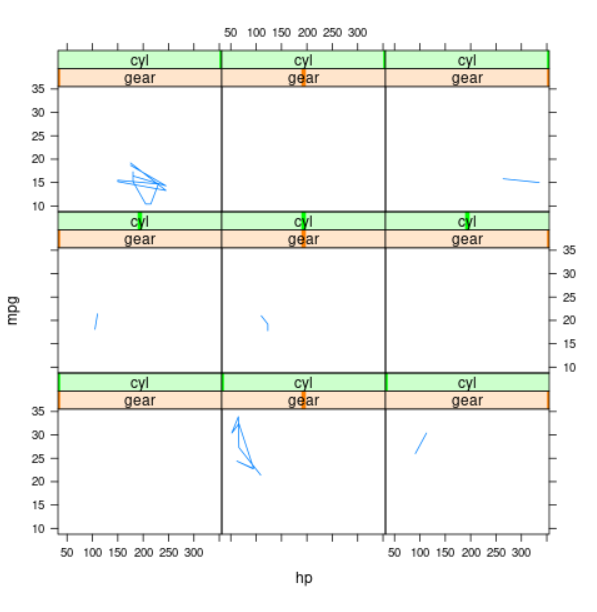### Mosaic Plot

A mosaic plot is a type of lattice graph that shows the distribution of two or more categorical variables. It is similar to a trellis graph, but it shows the proportion of observations in each category rather than the actual observations. For example, in R, we can create a mosaic plot using the “vcd” package and the “mosaic” function. Here is an example:

## R

 `library``(vcd)``data``(HairEyeColor)``mosaic``(HairEyeColor, shade = ``TRUE``)`

Output: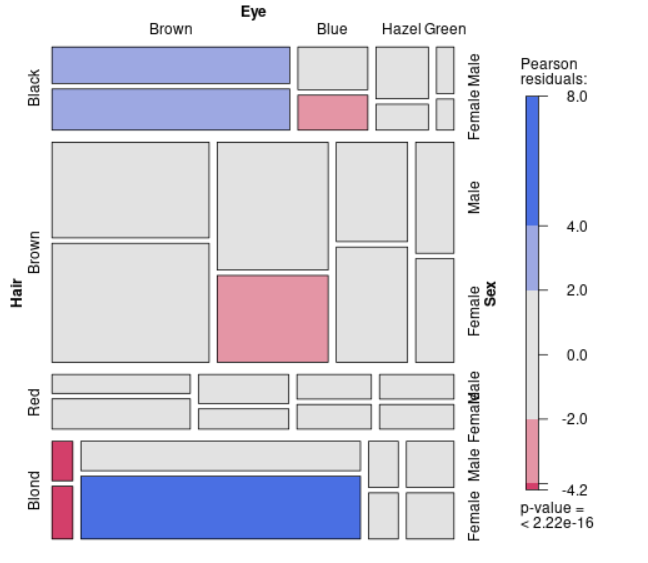### Dendrogram

A dendrogram is a type of lattice graph that shows the hierarchical structure of a dataset. It is used to represent the results of hierarchical clustering or other forms of data grouping. For example, in R, we can create a dendrogram using the “stats” package and the “hclust” function. Here is an example:

## R

 `library``(stats)``data``(mtcars)`` ` `d <- ``dist``(mtcars[, 1:4])``fit <- ``hclust``(d)``plot``(fit)`

Output: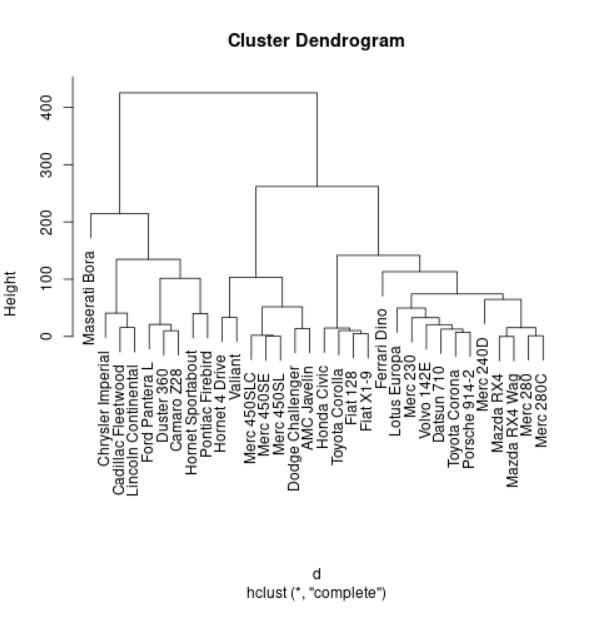### Countourplot:

In R, the ‘contour()’ function in the base graphics package can be used to create a contour plot, which is a graphical representation of a three-dimensional surface using a set of contour lines. The function takes in several parameters, including the x and y coordinates of the data points, the z-values, and the number of contour lines to be plotted. For example, the following code creates a contour plot of the peaks function:

## R

 `x <- ``seq``(-3, 3, length.out = 100)``y <- ``seq``(-3, 3, length.out = 100)``z <- ``outer``(x, y, ``function``(x, y) {``    ``r <- ``sqrt``(x^2 + y^2)``    ``10 * ``sin``(r) / r``})``contour``(x, y, z, nlevels = 15)`

Output: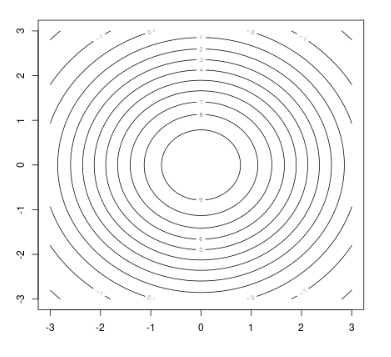### Stripplot:

A strip plot, also known as a one-dimensional scatter plot or a “stripchart”, is a type of plot that displays individual observations on a one-dimensional scale, usually along the x-axis. Each observation is represented by a small tick mark, or “jitter”, on the x-axis.

‘stripchart’ function from the ‘stats’ package: This function allows you to create a simple stripplot by providing a vector or data frame of values. Here’s an example of how to use it:

## R

 `library``(stats)`` ` `# create data``data <- ``rnorm``(1000, mean = 50, sd = 10)`` ` `# create stripplot``stripchart``(data, method = ``"jitter"``, pch = 20, col = ``"blue"``)`

Output: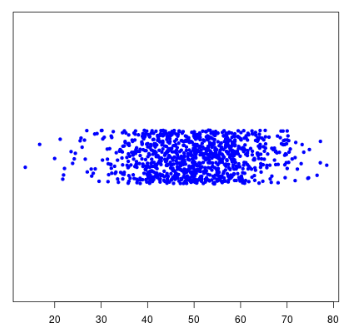### Barchart:

A bar chart, also known as a bar graph, is a chart that uses bars to represent different categories of data and the values associated with those categories. The length of each bar represents the magnitude of the value it represents. Bar charts can be used to display data in a variety of ways, including comparing different categories, showing changes over time, and displaying the distribution of data.

To create a barchart in R, you will need to use the ggplot2 library. In this example, the data is a data frame with two columns, “x” and “y”, representing the categories and values respectively. The ggplot function is used to create the barchart, with the data and aesthetic mapping (aes) specified. The geom_bar function is used to create the bars and the stat = “identity” argument is used to ensure that the bars are plotted at the correct height according to the values in the data. The fill argument is used to set the color of the bars. Additional arguments, such as xlab, ylab, and ggtitle, are used to add labels and a title to the chart.

## R

 `# Load ggplot2 library``library``(ggplot2)`` ` `# Create sample data``data <- ``data.frame``(x = ``c``(``"A"``, ``"B"``, ``"C"``, ``"D"``), ``                   ``y = ``c``(10, 20, 30, 40))`` ` `# Create barchart``ggplot``(data, ``aes``(x = x, y = y)) +``  ``geom_bar``(stat = ``"identity"``, fill = ``"blue"``) +``  ``xlab``(``"Categories"``) +``  ``ylab``(``"Values"``) +``  ``ggtitle``(``"Barchart Example"``)`

Output: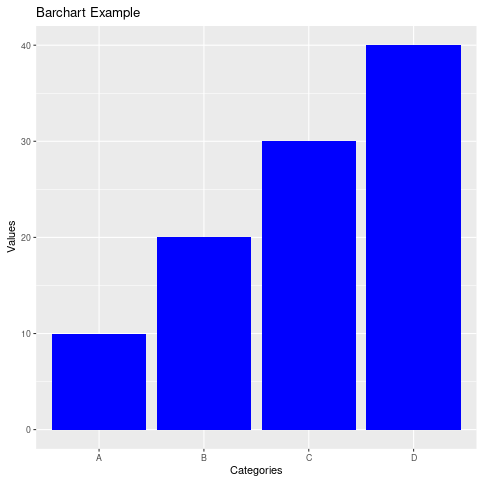### Density plot:

A density plot in R is a graphical representation of the distribution of a continuous variable. It shows the probability density function (PDF) of the variable, which is a smooth curve that represents the likelihood of a given value occurring.

To create a density plot in R, we can use the density() function from the base R package. This function takes a single vector of data as an argument and returns a plot of the density of the data.

## R

 `library``(ggplot2)`` ` `# Create some sample data``set.seed``(123)``data <- ``data.frame``(x = ``rnorm``(1000))`` ` `# Create the density plot``ggplot``(data, ``aes``(x = x)) + ``  ``geom_density``()`

Output:My Personal Notes arrow_drop_up